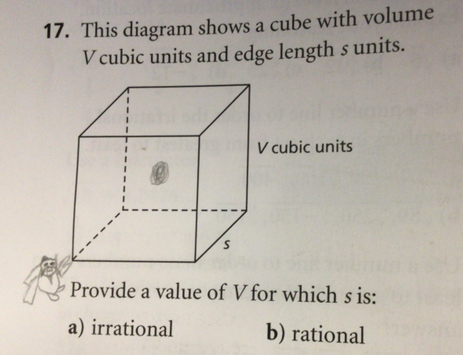Question# The graph below expresses a radical function that can be written in the form f(x) = a(x + k)^(1/n) + c. What does the graph tell you about the value of c in this function?

Functions
ANSWEREDThe graph below expresses a radical function that can be written in the form f(x) = a(x + k)^(1/n) + c. What does the graph tell you about the value of c in this function?